Courses

# Olympiad Test : Figures Puzzles -1

## 15 Questions MCQ Test National Cyber Olympiad Class 7 | Olympiad Test : Figures Puzzles -1

Description
This mock test of Olympiad Test : Figures Puzzles -1 for Class 7 helps you for every Class 7 entrance exam. This contains 15 Multiple Choice Questions for Class 7 Olympiad Test : Figures Puzzles -1 (mcq) to study with solutions a complete question bank. The solved questions answers in this Olympiad Test : Figures Puzzles -1 quiz give you a good mix of easy questions and tough questions. Class 7 students definitely take this Olympiad Test : Figures Puzzles -1 exercise for a better result in the exam. You can find other Olympiad Test : Figures Puzzles -1 extra questions, long questions & short questions for Class 7 on EduRev as well by searching above.
QUESTION: 1

### In this type of questions, a figure or a matrix is given in which some numbers are filled according to a rule. A place is left blank. You have to find out a character (a number or aletter) from the given possible answers which may be filled in the blank space. Q. Which one will replace the question mark?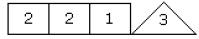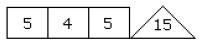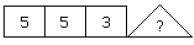Solution:

(2 × 2 – 1) = 3
and (5 × 4 – 5) = 15
(5 × 5 – 3) = 22

QUESTION: 2

### In this type of questions, a figure or a matrix is given in which some numbers are filled according to a rule. A place is left blank. You have to find out a character (a number or aletter) from the given possible answers which may be filled in the blank space. Q. Which one will replace the question mark?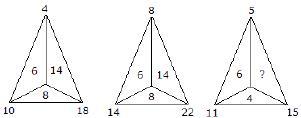Solution:

For first triangle,
10 – 4 = 6
18 – 10 = 8
18 – 4 = 14
For second triangle,
14 – 8 = 6
22 – 14 = 8
22 – 8 = 14
For third triangle,
11 – 5 = 6
15 – 11 = 4
15 – 5 = 10

QUESTION: 3

### In this type of questions, a figure or a matrix is given in which some numbers are filled according to a rule. A place is left blank. You have to find out a character (a number or aletter) from the given possible answers which may be filled in the blank space. Q. Which one will replace the question mark?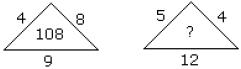Solution:

(4 + 8) × 9 = 108
(5 + 4) × 12 = 108

QUESTION: 4

In this type of questions, a figure or a matrix is given in which some numbers are filled according to a rule. A place is left blank. You have to find out a character (a number or aletter) from the given possible answers which may be filled in the blank space.
Q. Which one will replace the question mark?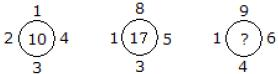Solution:

1 + 2 + 3 + 4 = 10
and 1 + 3 + 5 + 8 = 17
Similarly, 1 + 4 + 6 + 9 = 20

QUESTION: 5

In this type of questions, a figure or a matrix is given in which some numbers are filled according to a rule. A place is left blank. You have to find out a character (a number or aletter) from the given possible answers which may be filled in the blank space.
Q. Which one will replace the question mark?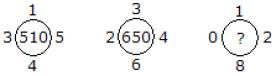Solution:

(1)2 + (5)2 + (4)2 + (3)2 = 51 × 10 = 510
and (3)2 + (4)2 + (6)2 + (2)2 = 65 × 10 = 650
Similarly, (0)2 + (1)2 + (2)2 + (8)2 = 69 × 10
= 690

QUESTION: 6

In this type of questions, a figure or a matrix is given in which some numbers are filled according to a rule. A place is left blank. You have to find out a character (a number or aletter) from the given possible answers which may be filled in the blank space.
Q. Which one will replace the question mark?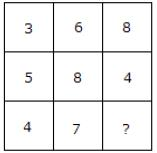Solution:

(5 + 3)/2 = 4
and (6 + 8)/2 = 7
Therefore (8 + 4)/2 = 6

QUESTION: 7

In this type of questions, a figure or a matrix is given in which some numbers are filled according to a rule. A place is left blank. You have to find out a character (a number or aletter) from the given possible answers which may be filled in the blank space.
Q. Which one will replace the question mark?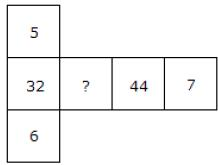Solution:

(5 × 6) + 2 = 32
(7 × 6) + 2 = 44
(7 × 5) + 2 = 37

QUESTION: 8

In this type of questions, a figure or a matrix is given in which some numbers are filled according to a rule. A place is left blank. You have to find out a character (a number or aletter) from the given possible answers which may be filled in the blank space.
Q. Which one will replace the question mark?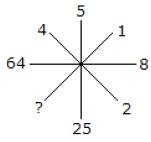Solution:

(2)2 = 4
(8)2 = 64
(5)2 = 25
(1)2 = 1

QUESTION: 9

In this type of questions, a figure or a matrix is given in which some numbers are filled according to a rule. A place is left blank. You have to find out a character (a number or aletter) from the given possible answers which may be filled in the blank space.
Q. Which one will replace the question mark?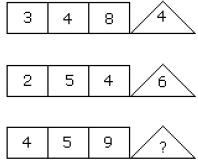Solution:

(3 × 4 – 8) = 4
(2 × 5 – 4) = 6
(4 × 5 – 9) = 11

QUESTION: 10

In this type of questions, a figure or a matrix is given in which some numbers are filled according to a rule. A place is left blank. You have to find out a character (a number or aletter) from the given possible answers which may be filled in the blank space.
Q. Which one will replace the question mark?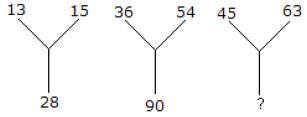Solution:

13 + 15 = 28
36 + 54 = 90
Therefore, 45 + 63 = 108

QUESTION: 11

In this type of questions, a figure or a matrix is given in which some numbersare filled according to a rule. A place is left blank.You have to find out a character (a number or aletter) from the given possible answers which maybe filled in the blank space.
Q. Which one will replace the question mark?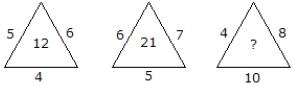Solution:

(5 × 6 × 4)/10 = 12
and (6 × 7 × 5)/10 = 21
Therefore (4 × 8 × 10)/10 = 32

QUESTION: 12

In this type of questions, a figure or a matrix is given in which some numbers are filled according to a rule. A place is left blank. You have to find out a character (a number or aletter) from the given possible answers which may be filled in the blank space.
Q. Which one will replace the question mark?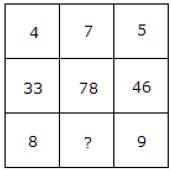Solution:

(4 × 8) + 1 = 33
(5 × 9) + 1 = 46
Similarly, (7 × 11) + 1 = 78

QUESTION: 13

In this type of questions, a figure or a matrix is given in which some numbers are filled according to a rule. A place is left blank. You have to find out a character (a number or aletter) from the given possible answers which may be filled in the blank space.
Q. Which one will replace the question mark?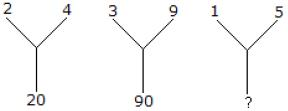Solution:

(2)2 + (4)2 = 20
(3)2 + (9)2 = 90
Therefore, (1)2 + (5)2 = 26

QUESTION: 14

In this type of questions, a figure or a matrix is given in which some numbers are filled according to a rule. A place is left blank. You have to find out a character (a number or aletter) from the given possible answers which may be filled in the blank space.
Q. Which one will replace the question mark?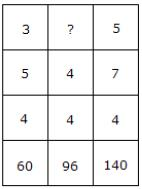Solution:

3 × 5 × 4 = 60
and 5 × 7 × 4 = 140
Therefore, 4 × 4 × ? = 96
? = (96/16) = 6.

QUESTION: 15

In this type of questions, a figure or a matrix is given in which some numbers are filled according to a rule. A place is left blank. You have to find out a character (a number or aletter) from the given possible answers which may be filled in the blank space.
Q. Which one will replace the question mark?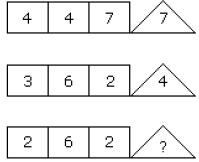Solution:

(4 × 7) ÷ 4 = 7
and (6 × 2) ÷ 3 = 4
Therefore, (6 × 2) ÷ 2 = 6